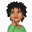RELATED TAGS

# How to sort a list of tuples in Python using LambdaVao11

### Overview

When we sort a list with a lambda function, it enables the creation of custom sorting orders. The sort() method and sorted() function can sort lists in descending or ascending (alphabetical and numerical) order by default.

### Syntax

1. sort(): This is a built-in list method called list.sort(). The sort() method sorts the list in place. It updates the order of the original list.

list.sort(key = none, reverse = false)

2. sorted(): This sorted() function sorts a data collection like a list and returns a new sorted list. This function sorts the list and creates a new list.

list.sorted(iterable, key function, reverse)


### What is a list?

A list is an ordered and mutable collection data type that allows duplicates. Lists are suitable for data that needs to be modified.

#### How to create a list in Python

A list is declared using square brackets ([]).

Below are examples of different types of lists:

• An empty list.
• A mixed list with different data types. The example below contains string, integer, float, and boolean values.
• A nested list with different data collection types, a list and a tuple.
• A nested list of tuples. This will be sorted using the sort() method and sorted() function with the lambda function.
emptyList= []
print(emptyList)
print(type(emptyList))

### What is a tuple?

A tuple is an ordered collection data type that allows duplicates. A tuple allows multiple values to be assigned to one variable. A tuple is good for data access as the values are fixed in a tuple once declared.

#### How to create a tuple in Python

A tuple is declared using parentheses ().

• An empty tuple.
• A mixed tuple with different data types. The example below contains string, integer, float, and boolean values.
• A nested tuple with different data collection types, a list and a tuple.
emptyTuple = ()
print(emptyTuple)
print(type(emptyTuple))
Creating tuples in three ways

Lambda: It is an anonymous function. This means we do not need to declare the function as def before using it.

Key: It is a keyword argument that can accept any function as a value.

Iteratable: It is a data collection object that can be looped through in a sequence, similar to a list, tuple, or dictionary.

Parts of tuple syntax

### List of tuples

We’ll use the planet list of tuples in the following example:

//List of Tuples Declaration
planets = [("Earth", 3),("Jupiter",5),("Mercury",1), ("Mars",4), ("Neptune",8), ("Saturn", 6), ("Uranus",7), ("Venus",2)]
print(planets)


### Lambda sorting examples

The following examples will be used to illustrate how lambda functions can enhance or extend the functionality of the list.sort() method and sorted() function using the key parameter.

#### Example 1: sorted() function

The sorted() function takes the planets list as data, the lambda sets the function key parameter. The list is sorted on the second element of the tuple which is the number, and the reverse parameter is set to true.

newList = sorted(planets, key = lambda x : x,reverse=True)
print("Reverse sorted list: ",newList)
print("original list: ", planets)


#### Output:

This output shows the lambda function change the default sorted() function parameter values.

The sorted() function returns a new list object, assigned to the newList variable. The newList is listed in reverse numerical descending order.

The original list is not changed.

('Reverse sorted list: ', [('Neptune', 8), ('Uranus', 7), ('Saturn', 6), ('Jupiter', 5), ('Mars', 4), ('Earth', 3), ('Venus', 2), ('Mercury', 1)])

('Original list: ', [('Earth', 3), ('Jupiter', 5), ('Mercury', 1), ('Mars', 4), ('Neptune', 8), ('Saturn', 6), ('Uranus', 7), ('Venus', 2)])


#### Example 2: list.sort() method

The list.sort() method key parameter is set to lambda. The arguement x is the iterable element (tuple) to be sorted by the second element, the number.

The lambda expression sorts the list by the second element of the tuple value and updates the original. The updated list is not returned.

planets.sort(key = lambda x : x)


The in-place sort returns a value of none but the original list is sorted.

print("In-Place Sort: ",planets.sort(key = lambda x : x))

//print sorted list
print(planets)


#### Output:

The output displays the result of the lambda expression passed in for the key parameter of list.sort():

• Printing the planets list shows it is updated, and sorted in ascending order of the second element of the tuple.
    ('In-Place Sort: ', None)

('Updatd List: ', [('Mercury', 1), ('Venus', 2), ('Earth', 3), ('Mars', 4), ('Jupiter', 5), ('Saturn', 6), ('Uranus', 7), ('Neptune', 8)])


Try out the code in the console below:

# List of Tuples Declaration
planets = [("Earth", 3),("Jupiter",5),("Mercury",1), ("Mars",4), ("Neptune",8), ("Saturn", 6), ("Uranus",7), ("Venus",2)]
print(planets)

newList = sorted(planets, key = lambda x : x,reverse=True)
print("Sorted List: ",newList)
print("original List: ", planets)

# Sorts the list by the item value of the second element of the Tuple
SelectPlanets = sorted(planets, key = lambda x : x)

print(SelectPlanets)
Sorting a list of tuples with the sorted() function and the list.sort() method

RELATED TAGS

CONTRIBUTORVao11
RELATED COURSES

View all Courses

Keep Exploring

Learn in-demand tech skills in half the time